# Applied Mathematics Faculty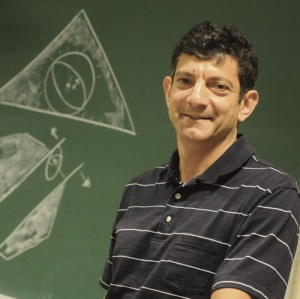Daniel Bienstock Applied mathematics, methodology and high-performance implementation of optimization algorithms, applications of optimization: preventing national-scale blackouts, emergency management, approximate solution of massively large optimization problems, higher-dimensional reformulation techniques for integer programming, robust optimizationQiang Du Numerical analysis, mathematical modeling and scientific computation with selected applications in physical, biological, materials, data and information sciences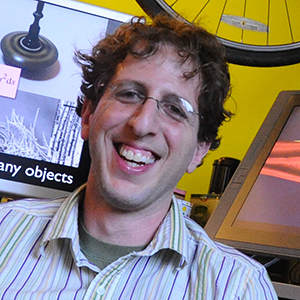Eitan Grinspun Computer graphics and scientific computing, computational mechanics, mathematical foundations of graphics, discrete differential geometry, computer science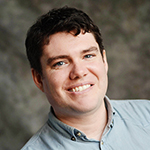Kyle Mandli Computational and analytical aspects of geophysical problems dealing with shallow mass; Development of adaptive mesh re finement strategies for geophysics; Design and implementation of wave propagation softwareLorenzo M. Polvani Atmospheric and climate dynamics, geophysical fluid dynamics, numerical methods for weather and climate modeling, planetary atmospheresNumerical analysis, scientific computation, applied analysis and partial differential equations, inverse problems and imaging, random graphs and networks, kinetic modeling and simulationsAmir Sagiv Differential equations, dynamics, optics, uncertainty quantification, approximation theory, numerical analysis, and mathematical analysis.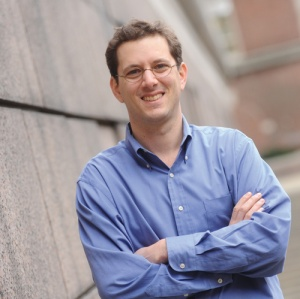Adam H. Sobel Atmospheric and climate dynamics, tropical meteorology, extreme weather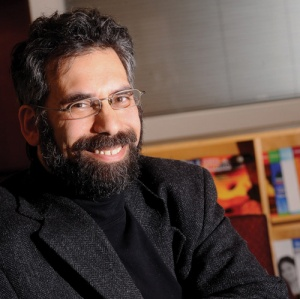Marc W. Spiegelman Advanced computation for multi-physics problems with applications to coupled fluid-solid mechanics in Earth Sciences (e.g. magma dynamics, carbon sequestration)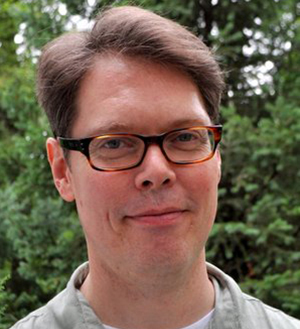Predictability and variability of the climate system, with emphasis on the application of statistical methods to data from observations and numerical models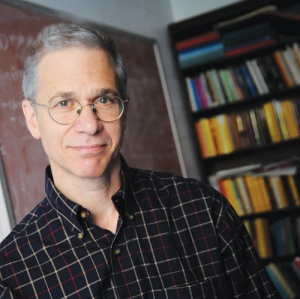Michael I. Weinstein Applied and fundamental mathematics, partial differential equations, multi-scale analysis, dynamical systems; waves in nonlinear, inhomogeneous and random media; applications to optics and photonics, quantum and fluid systems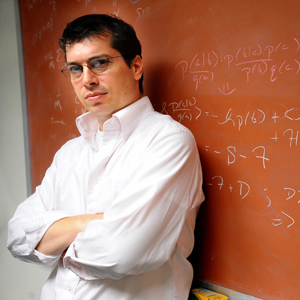Chris H. Wiggins Applied mathematics, mathematical biology, biopolymer dynamics, soft condensed matter, genetic networks and network inference, machine learningDrew Youngren Microlocal Analysis, Partial Differential Equations, Mathematics Education

500 W. 120th St., Mudd 200, MC 4701 New York, NY 10027 / Phone: 212-854-4457 / Fax: 212-854-8257 / Email: apam@columbia.edu